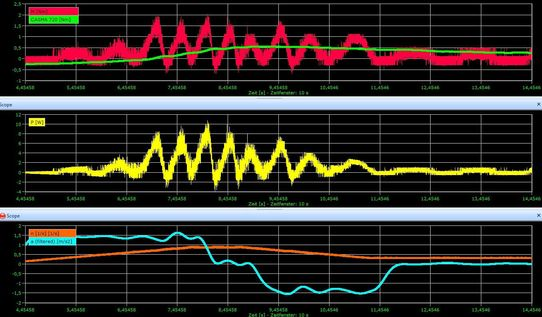# Tips & Tricks on Using the CASMA Filter for Your Torque Measurements

The torque signal in tests of combustion engines exhibits dynamic behavior. The reason for this is vibrations and oscillations caused by the working cycles of each individual cylinder. These dynamic, periodically oscillating torque components are superimposed over the actual torque measurement.

The CASMA filter (Crank Angle Sampled Moving Average) works in angular synchronism and is time independent, thus allowing to automatically respond to RPM changes. This filter does not work in the time domain. Instead it works synchronously to the angle.

In this article you will learn how to apply the CASMA filter in HBM's TIM-PN, TIM-EC and PMX signal conditioning systems.

## Filtering the torque signal

### Time-synchronous filters

To ensure reliable control, the noisy signal must be filtered or smoothed. Filters such as Bessel or Butterworth work time-synchronously with low-pass characteristics.

### Angular filters

The CASMA filter (Crank Angle Sampled Moving Average) works in angular synchronism and is time independent, thus allowing to automatically respond to RPM changes.

## How to use the CASMA filter in TIM-PN and TIM-EC

### Web Interface

The TIM-EC and TIM-PN torque interface modules offer an integrated web server. In this browser-based software you can use all of the relevant settings for using the CASMA filter.

The required filter parameters are entered via the HTML page and checked for plausibility. The entry point to the CASMA filter is integrated into the "Units and filters" menu.

### Features of the CASMA Filter

#### Parameterization Characteristic

 Parameterization characteristic Function Angle divider Reduces the angle resolution, thus allowing higher rotational speeds in the same window width Angle range (degrees) Angle range (window width) over which the moving average operates Pseudo speed (rpm) After the rpm speed, pseudo pulses or a pseudo speed are generated. Otherwise the filter would stop working and the measured value would freeze.

#### Information Area Feature

 Information area feature Function Maximum rotational speed (rpm) The angle pulses/s must be less than the sampling rate of the torque measurement. Otherwise the average would be formed via the same measured value. Pulses per revolution Comes from the number of increments, and the analysis. With active quadrature analysis, the angular resolution is quadrupled Angular resolution in degrees Calculated from the number of pulses per revolution and the divider Number of averaged values Computes the number of measured values used to form the moving average

## How to use the CASMA filter with PMX

### Create a calculated channel

Create a new calculated channel “rotation synchronous filter” in the Analysis category.• Filter Input: Enter the signal to be filtered here.
• Shaft Angle Input: Enter the signal of the angle of rotation sensor here. The measured values must be between 0° and 360°.
• Window Width: Specify the range for taking the moving average. The width must be between 30° and 720°. The default setting is 180°. The ratio of the window width to the resolution must be less than 180.

The window width can be easily determined experimentally by projecting the periodic time on the disturbance to the angle of rotation (see screenshot). In this example, there is a periodic disturbance every 720°.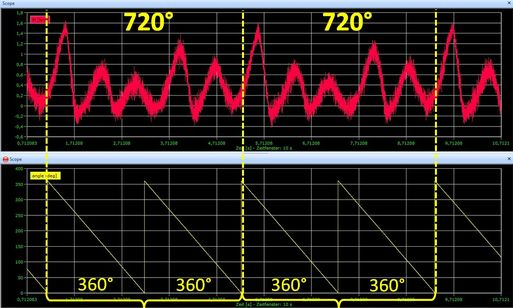• Minimum Speed: This virtual rotational speed is applied when the active rotational speed is less than the defined minimum rotational speed.
• Resolution: This value determines how often (how many degrees each time) a new average will be calculated. Note that the maximum permitted permissible rotational speed depends on this value because the speed of calculation is determined by the overall update rate.

The theoretical value is derived by: Maximum rotational speed = resolution * overall update rate / 6.

For practical purposes you should use values that amount to only 10 to 20% of this theoretically possible maximum rotational speed.

 Resolution The theoretical maximum rotational speed at an overall update rate of 19,200 Hz The theoretical maximum rotational speed at an overall update rate of 38,400 Hz 1° 3200 rpm 6400 rpm 2° 6400 rpm 12,800 rpm 4° 12,800 rpm 25,600 rpm 6° 19,200 rpm 38,400 rpm 8° 25,600 rpm 51,200 rpm

The following multiples of the rotational speed are suppressed depending on the window width:

 Window width Multiples 90° 4, 8, 12, … 120° 3, 6, 9, … 180° 2, 4, 6, … 360° 1, 2, 3, … 720° 0, 5, 1, 1, 5, …

Note: If one of the source signals is invalid, the output signal will be invalid as well.

### CASMA in action

You will see the unfiltered torque signal which is red, and the filter torque signal filtered using CASMA in green.

It can be seen clearly that the CASMA filter achieves excellent stabilization of torque measurements in correlation to the engine speed, which also changes over time. The greater the width of this filter, the better the results.

### Example

In this short example, PMX is used for analyzing power P[W], rotational speed n [1/s] and acceleration [1/s²], in addition to torque M [Nm] and the corresponding CASMA filter. The calculated channels as shown in the screenshot below have been created for this purpose. The signals are displayed in catman.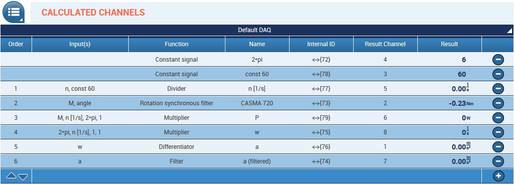### Calculations:

Power: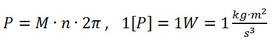Angular speed: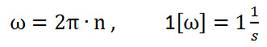Angular acceleration: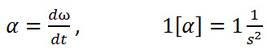Important: For all calculations the rotation speed n has to be converted from revolutions per minute to revolutions per second, i.e. divided by 60.

### Channels

Please see below for all channels displayed graphically in catman. The colors in brackets refer to the colors of the curves. The signals consist of the following channels:
Torque M (red):

• Directly from the measurement channel of the PX460

CASMA filter (green):

Power P (yellow):

• Divider (rotational speed n / 60), revolutions per second
• Constant signal (2*pi)
• Multiplier (M * n [1/s] * 2*pi]

Rotational speed n (orange):

• Directly from the measurement channel of the PX460

Angular acceleration (blue):

• Multiplier (2*pi * n [1/s]), results in the angular speed w
• Differentiator (w)